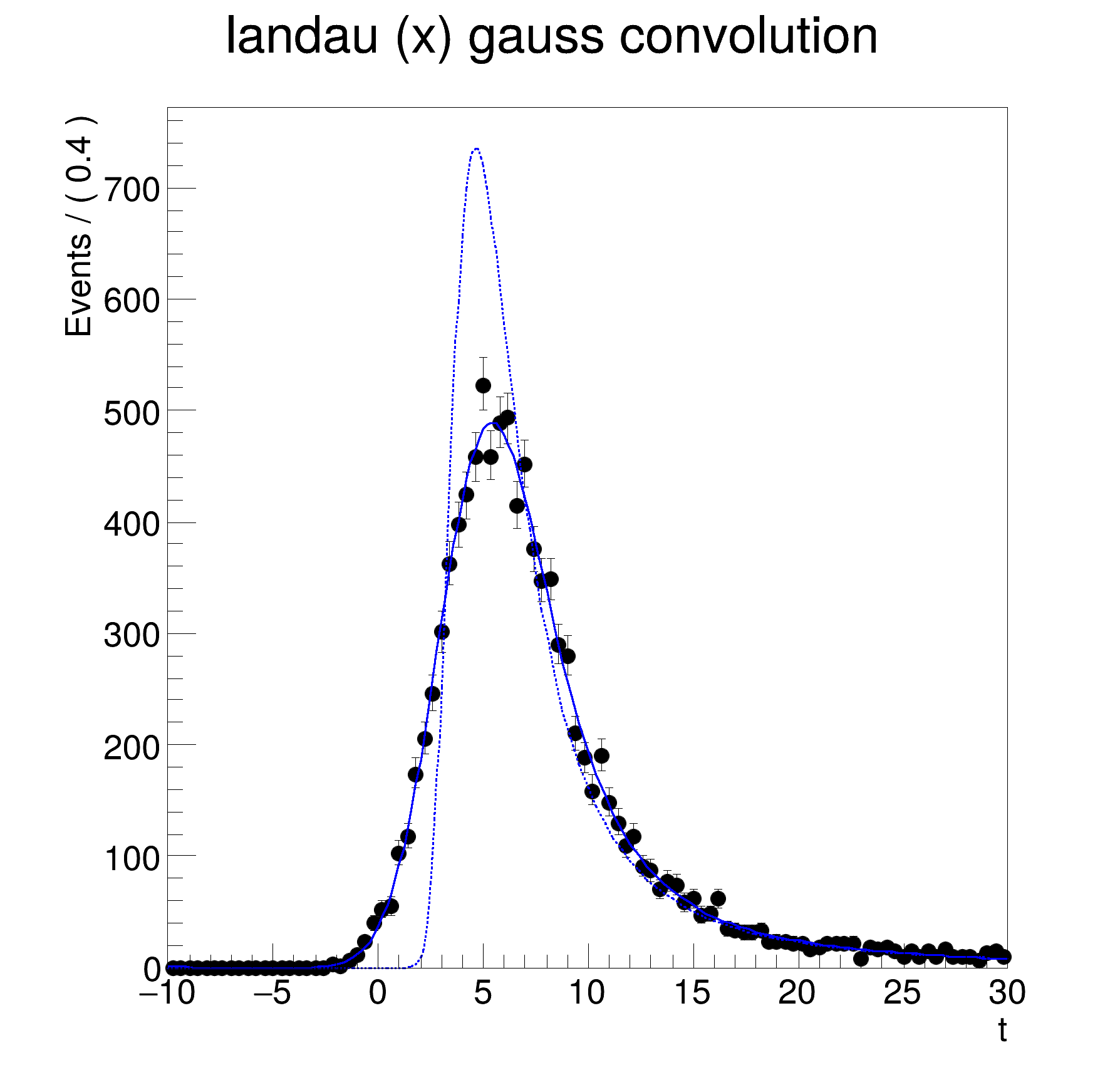ROOT   Reference Guide
Searching...
No Matches
rf208_convolution.C File Reference

## Detailed DescriptionAddition and convolution: one-dimensional numeric convolution

pdf = landau(t) (x) gauss(t)
Double_t x[n]
Definition legend1.C:17

This tutorial requires FFT3 to be enabled.#include "RooRealVar.h"
#include "RooDataSet.h"
#include "RooGaussian.h"
#include "RooLandau.h"
#include "RooFFTConvPdf.h"
#include "RooPlot.h"
#include "TCanvas.h"
#include "TAxis.h"
#include "TH1.h"
using namespace RooFit;
{
// S e t u p c o m p o n e n t p d f s
// ---------------------------------------
// Construct observable
RooRealVar t("t", "t", -10, 30);
// Construct landau(t,ml,sl) ;
RooRealVar ml("ml", "mean landau", 5., -20, 20);
RooRealVar sl("sl", "sigma landau", 1, 0.1, 10);
RooLandau landau("lx", "lx", t, ml, sl);
// Construct gauss(t,mg,sg)
RooRealVar mg("mg", "mg", 0);
RooRealVar sg("sg", "sg", 2, 0.1, 10);
RooGaussian gauss("gauss", "gauss", t, mg, sg);
// C o n s t r u c t c o n v o l u t i o n p d f
// ---------------------------------------
// Set #bins to be used for FFT sampling to 10000
t.setBins(10000, "cache");
// Construct landau (x) gauss
RooFFTConvPdf lxg("lxg", "landau (X) gauss", t, landau, gauss);
// S a m p l e , f i t a n d p l o t c o n v o l u t e d p d f
// ----------------------------------------------------------------------
// Sample 1000 events in x from gxlx
std::unique_ptr<RooDataSet> data{lxg.generate(t, 10000)};
// Fit gxlx to data
lxg.fitTo(*data, PrintLevel(-1));
// Plot data, landau pdf, landau (X) gauss pdf
RooPlot *frame = t.frame(Title("landau (x) gauss convolution"));
data->plotOn(frame);
lxg.plotOn(frame);
landau.plotOn(frame, LineStyle(kDashed));
// Draw frame on canvas
new TCanvas("rf208_convolution", "rf208_convolution", 600, 600);
frame->GetYaxis()->SetTitleOffset(1.4);
frame->Draw();
}
@ kDashed
Definition TAttLine.h:48
Option_t Option_t TPoint TPoint const char GetTextMagnitude GetFillStyle GetLineColor GetLineWidth GetMarkerStyle GetTextAlign GetTextColor GetTextSize void data
PDF for the numerical (FFT) convolution of two PDFs.
Plain Gaussian p.d.f.
Definition RooGaussian.h:24
Landau distribution p.d.f.
Definition RooLandau.h:24
A RooPlot is a plot frame and a container for graphics objects within that frame.
Definition RooPlot.h:43
static RooPlot * frame(const RooAbsRealLValue &var, double xmin, double xmax, Int_t nBins)
Create a new frame for a given variable in x.
Definition RooPlot.cxx:239
TAxis * GetYaxis() const
Definition RooPlot.cxx:1279
void Draw(Option_t *options=nullptr) override
Draw this plot and all of the elements it contains.
Definition RooPlot.cxx:652
RooRealVar represents a variable that can be changed from the outside.
Definition RooRealVar.h:37
virtual void SetTitleOffset(Float_t offset=1)
Set distance between the axis and the axis title.
Definition TAttAxis.cxx:298
The Canvas class.
Definition TCanvas.h:23
The namespace RooFit contains mostly switches that change the behaviour of functions of PDFs (or othe...
[#1] INFO:Eval -- RooRealVar::setRange(t) new range named 'refrange_fft_lxg' created with bounds [-10,30]
[#1] INFO:Caching -- RooAbsCachedPdf::getCache(lxg) creating new cache 0x563261cee6a0 with pdf lx_CONV_gauss_CACHE_Obs[t]_NORM_t for nset (t) with code 0
[#1] INFO:Minimization -- RooAbsMinimizerFcn::setOptimizeConst: activating const optimization
[#1] INFO:Minimization -- RooAbsMinimizerFcn::setOptimizeConst: deactivating const optimization
[#1] INFO:Caching -- RooAbsCachedPdf::getCache(lxg) creating new cache 0x563264548430 with pdf lx_CONV_gauss_CACHE_Obs[t]_NORM_t for nset (t) with code 0
Date
July 2008

Definition in file rf208_convolution.C.